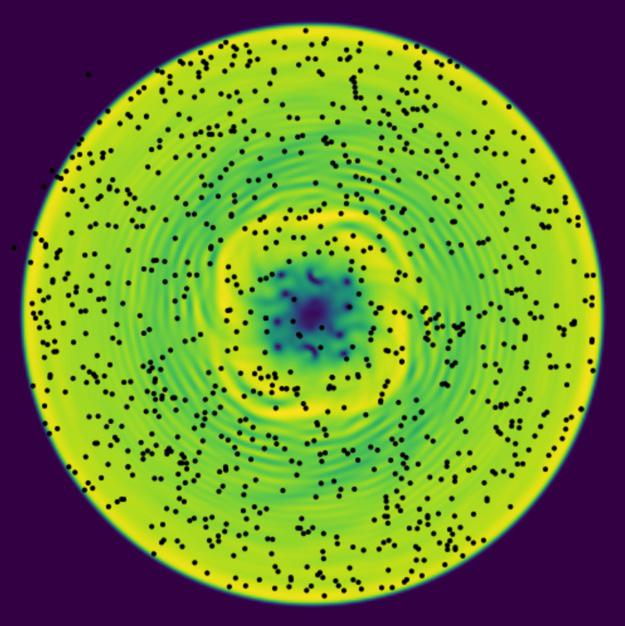Department of Physics and Astronomy

The Forbes Group

super_hydro: Superfluid Hydrodynamics Explorer## super_hydro: Exploring Superfluids¶

Nobel laureate Richard Feynman said: "I think I can safely say that nobody really understands quantum mechanics". Part of the reason is that quantum mechanics describes physical processes in a regime that is far removed from our every-day experience – namely, when particles are extremely cold and move so slowly that they behave more like waves than like particles.

This application attempts to help you develop an intuition for quantum behavior by exploiting the property that collections of extremely cold atoms behave as a fluid – a superfluid – whose dynamics can be explored in real-time. By allowing you to interact and play with simulations of these superfluids, we hope you will have fun and develop an intuition for some of the new features present in quantum systems, taking one step closer to developing an intuitive understanding of quantum mechanics.

Beyond developing an intuition for quantum mechanics, this project provides an extensible and general framework for running interactive simulations capable of sufficiently high frame-rates for real-time interactions using high-performance computing techniques such as GPU acceleration. The framework is easily extended to any application whose main interface is through a 2D density plot - including many fluid dynamical simulations.

### Intuitive Quantum Mechanics¶

Because we interact with objects at room temperature, our intuition is based on the behavior of objects at this scale, which is well described by the laws of classical mechanics as formulated by Newton. Our intuition starts to fail when we move away from this regime, either at speeds comparable to that of light, at which point Einstein's theory of relativity takes over and we must give up our notion of time moving at the same rate for everyone, or at very slow speeds where quantum mechanics takes over.

This application takes advantage of a hydrodynamic formulation of quantum mechanics that allows you to directly visualize and explore the behaviour of quantum fluids – superfluids – to develop an intuition for what happens in states of ultra-cold matter such as can be found in cold-atom laboratories around the world (such as the Fundamental Quantum Physics Lab at WSU), and in neutron stars, where the neutrons form a superfluid (cold compared to relevant nuclear scales).

## Demo¶

Embedded Movie of various demo.

### Installing¶

The easiest way to install the super_hydro explorer is to use Conda and then run it using the Jupyter notebook interface:

conda env create mforbes/super_hydro
conda activate super_hydro
# conda install cupy  # Enable GPU support if supported.
# pip install cupy    # If no conda image exists yet for your platform...


This will create a Conda environment called super_hydro with everything you need to run the code.

## Superfluid Hydrodynamics¶

Superfluids – especially Bose-Einstein condensates (BECs) can be described quite accurately by the Gross-Pitaevskii equation (GPE), which is a form of non-linear Schrödinger equation:

$$\newcommand{\vect}{\mathbf{#1}} \newcommand{\abs}{\lvert#1\rvert} \newcommand{\I}{\mathrm{i}} \newcommand{\d}{\mathrm{d}} \newcommand{\pdiff}[]{\frac{\partial^{#1} #2}{\partial {#3}^{#1}}} \newcommand{\diff}[]{\frac{\d^{#1} #2}{\d {#3}^{#1}}} \I\hbar\pdiff{\Psi(x, t)}{t} = \left( -\frac{\hbar^2}{2m}\nabla^2 + V(x, t) + \overbrace{g\abs{\Psi(x, t)}^2}^{\text{non-linear term}} \right)\Psi(x, t).$$

Here $\Psi(x, t)$ is the complex condensate wavefunction and $n(x, t) = \abs{\Psi(x, t)}^2$ is the density of the superfluid at point $x$ and time $t$. At low densities, one can neglect the non-linear term and the condensate wave-function evolves exactly as a single quantum particle would behave. As the number of particles increases, however, they interact through this non-linear "mean-field" term, giving rise to many interesting non-linear phenomena such as sound waves (phonons), solitons, and vortices.

Understanding a complex-valued function like the wavefunction $\Psi(x, t)$ can be a challenge – it is not something most people have developed an intuition for. However, one can find a more relatable representation through what is called a Madelung transformation:

$$\Psi(x, t) = \sqrt{n(x, t)} e^{\I \phi(x, t)}, \qquad u(x, t) = \frac{\hbar}{m}\nabla\phi(x, t).$$

With this transformation, the GPE now has the following hydrodynamic form:

$$\pdiff{n(x, t)}{t} + \nabla\Bigl(n(x, t)u(x,t)\Bigr)= 0,\\ \diff{u(x, t)}{t} = \pdiff{u(x, t)}{t} + u(x, t) \cdot \nabla u(x, t) = -\frac{1}{m}\nabla\left( \overbrace{-\frac{\hbar^2}{2m}\frac{\nabla^2 \sqrt{n(x, t)}}{\sqrt{n(x, t)}}}^{\text{quantum potential}} + V(x, t) + g n(x, t) \right).$$

These equations describe a fluid with density $n(x, t)$ and flow velocity $u(x, t)$. All of the quantum effects now appear in the so-called quantum potential. Otherwise, these equations have exactly the same form as the Euler equations) which describe a perfect fluid.

Note: this description is exactly equivalent to the original GPE, but now allows us to interpret and visualize the quantum dynamics as a classical fluid. The super_hydro explorer provides a visualization for the two components: $n(x, t)$ as a background density image, and the flow $u(x, t)$ is represented by moving "tracer particles" which trace out the Bohmian trajectories.

## Funding¶This material is based upon work supported by the National Science Foundation under Grant Number 1707691. Any opinions, findings, and conclusions or recommendations expressed in this material are those of the author(s) and do not necessarily reflect the views of the National Science Foundation.